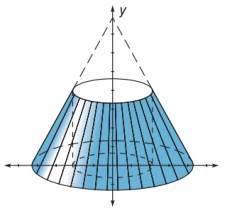Chapter 10.1, Problem 42EElementary Geometry For College St...

7th Edition
Alexander + 2 others
ISBN: 9781337614085

Solutions

Chapter
SectionElementary Geometry For College St...

7th Edition
Alexander + 2 others
ISBN: 9781337614085
Textbook Problem

Find the volume of the solid formed when the triangular region having vertices at 2, 0 , 4, 0 , and 2, 4 is rotated about the y -axis.To determine

To show:

The volume of solid when the triangular region having vertices 2, 0, 4, 0 and 2, 4 is rotated about the y-axis.

Explanation

A triangle is drawn with the following vertices 2, 0, 4, 0 and 2, 4.

Now, the triangle is rotated about the y-axis.

The resultant will be a cone.

Now, we have to find the volume of the cone.

Let h be the height of the cone.

Let r be the radius of the cone.

From the diagram,

Height is the distance between the points 2, 4 and 2, 0.

x1,y1=2, 4

x2,y2=2, 0

Formula for distance between two points

d=x2-x12+y2-y12

Substituting the x and y co-ordinates

d=2-22+0-42

On solving this,

d=02+-42

d=42

d=16

d=4

Hence, height is 4 units.

Radius is the distance between the points 2, 0 and 4, 0

Still sussing out bartleby?

Check out a sample textbook solution.

See a sample solution

The Solution to Your Study Problems

Bartleby provides explanations to thousands of textbook problems written by our experts, many with advanced degrees!

Get Started

Find more solutions based on key concepts